# Power Ratios

The decibel equivalent for any power ratio is

Equation 1.4where p 1 /p 2 is a power ratio.

In two circuits of equal impedance, the ratio of their powers may be determined as the square of the ratios of the voltages. This definition of a power ratio, in units of decibels, assumes equal impedances in the two circuits :

Equation 1.5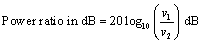where v 1 / v 2 is the ratio of RMS voltages across two circuit elements of equal resistance, or in the case of identical signal shapes in the two circuits, such as sine waves, the ratio of voltage amplitudes across two circuits having equal complex-valued impedances, and the same formula and conditions apply to the determination of a power ratio from a ratio of currents.

If the impedances in the two circuits differ , then the power ratio must be corrected for that difference.

Equation 1.6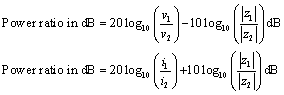where v 1 / v 2 is the ratio of RMS voltages across two circuit elements having resistances z 1 and z 2 respectively, or in the case of identical signal shapes in the two circuits, such as sine waves, the ratio of voltage amplitudes across two circuits having proportional complex-valued impedances. i 1 /i 2 is the ratio of RMS currents flowing through two circuit elements having resistances z 1 and z 2 respectively, or in the case of identical signal shapes in the two circuits, such as sine waves, the ratio of current amplitudes across two circuits having proportional complex-valued impedances.

In some cases it is convenient to use decibel notation to express a ratio of the voltages (or currents) within two circuits without consideration of the level of power.

Equation 1.7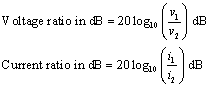where v 1 / v 2 is the ratio of voltage amplitudes within the two circuits. Presumably, the two circuits carry signals with identical shapes, such as sine waves. i 1 /i 2 is the ratio of current amplitudes within the two circuits. Presumably, the two circuits carry signals with identical shapes, such as sine waves.

The magnitude of a voltage, current, or power relative to a standard reference level may be expressed in absolute terms using decibel notation. For example, the notation dBmV refers to the following quantity:

Equation 1.8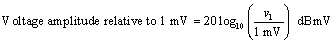where v 1 represents the voltage amplitude of a signal generally assumed to be sinusoidal.

Units of nepers occasionally appear in transmission-line problems. One neper equals 8.685889638065 dB. Definitions of power, voltage, and current ratios in units of nepers use the natural (base- e ) logarithm ln ().

Equation 1.9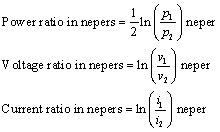where p 1 /p 2 is a power ratio, v 1 / v 2 is the ratio of voltage amplitudes within the two circuits. Presumably, the two circuits carry signals with identical shapes, such as sine waves. i 1 /i 2 is the ratio of current amplitudes within the two circuits. Presumably, the two circuits carry signals with identical shapes, such as sine waves. ln( x ) is the natural logarithm function (log base e of x ).

## Historical note

The term neper was named after John Neper, 1550 “1617, "Scottish mathematician and inventor of logarithms" ( Webster's Encyclopedic Unabridged Dictionary of the English Language , 1989). His name has been somewhat confused in history with that of Sir Charles James Napier, 1782 “1853, a British general and the inventor of a "diagram for showing the deviation of a magnetic compass from magnetic north at any heading" (ibid). The result today is that you may occasionally see the unit neper spelled napier . According to the American National Standards Institute, the correct spelling is neper . Further confusing the issue is that the correct spelling for the term that means "natural logarithm" is Napierian logarithm .

POINT TO REMEMBER

• One neper equals 8.685889638065 dB.High-Speed Signal Propagation[c] Advanced Black Magic
ISBN: 013084408X
EAN: N/A
Year: 2005
Pages: 163